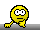## Group “effect” [Design Issues]

Hi GM,

» […] fixed effects used in general crossover studies and possible reasons for significant results of these fixed effects, I didn't found any relevant reasons for the significant group effect.

The p-value tells you only how likely something occurs. If you set your limit to 0.05 that means that you consider a p-value <0.05 denoting an effect which occurred not by pure chance. The reason for an effect is beyond the reach of statistics.
Think about the subject-term. It is always highly significant.* Recode the common effects to something neutral (say, response → Y, sequence → a, subject → b, period → c, treatment → d ) and provide the data to a statistician without telling the background to evaluate the linear model $$\ln(Y) \sim a+b+c+d$$ or – if you insist on the stupid over-specified one given in the guidelines – $$\ln(Y) \sim a+b(a)+c+d$$ Hey, $$p(b)$$ or $$p(b(a)) = 0.00000314$$. Now ask for a “reason”.
Answer:Science is wonderfully equipped to answer the question “How?”
but it gets terribly confused when you ask the question “Why?”
Erwin Chargaff

Ask a physicist what gravity is. No, not how it is described in physics. You will be surprised.

» Anybody know about the possible reasons for significant group effect...?

Chance? IMHO, testing for it is futile (see there).

• Unless you perform the study in cloned subjects, which would violate an assumption of the model, namely independence.If I would ever see a study with a p-value >0.05 for the subject effect it would ring my alarm bells.

Dif-tor heh smusma 🖖Helmut SchützThe quality of responses received is directly proportional to the quality of the question asked. 🚮
Science QuotesIng. Helmut Schütz